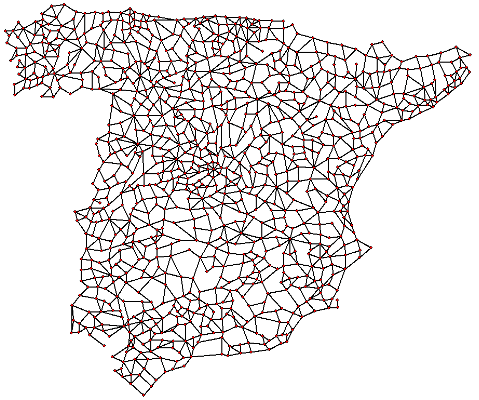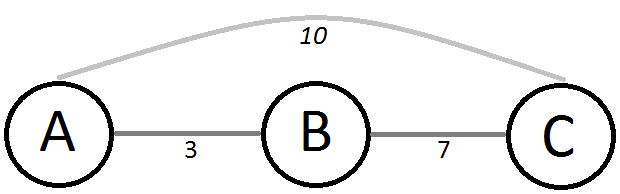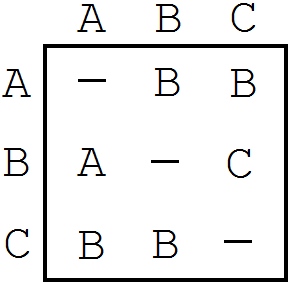# Sparse Travelling Salesman Problem

## Introduction

The Travelling Salesman Problem (TSP) is one of those few problems that caught my attention from the first moment. Probably because is a fairly simple concept, although there is a lot of complexity in it. Let’s remember the problem statement.

Given a list of cities and the distances between each pair of cities, what is the shortest possible route that visits each city exactly once and returns to the origin city?

This statement is rather easy to understand. However, it supposes that you can always move from one city to any other, and I’ve thought many times that this is not the most interesting approach for a Travelling Salesman.

The idea of every node connected with every node sounds sometimes too ideal. In lot of problems that will not be possible. If you are visiting cities maybe you can’t go from one city to another because there is a river or no road. For example this graph which represents a road map.Although is not necessary being so literal to notice that working with sparse graphs instead of complete graphs might be interesting. Moreover, in this variation, we should be able to repeat nodes to ensure that we find the optimal tour.

In this article, I will explain the Sparse Travelling Salesman Problem and an interesting approach to solve it.

## Overview

The main idea of this variation of the problem is that you may not be able go from a certain node to another directly, but you can go across other nodes.

However, this variaton adds some complexity because in the standard TSP you will always be able to go directly to a node that hasn’t been visited yet. Nevertheless, in the sparse variation you might reach a node where all the adjacent nodes have already been visited, so you have to return to one of the visited nodes to reach a node that haven’t been visited yet. That’s why you should be able to repeat nodes in the tour.

Alright, if you want to move between two non-adjacent nodes you should take the shortest path. For that task, you can use Dijkstra’s Algorithm. However, if you have to apply it for every node along the program execution it will have a big computational cost and it will add complexity to our tour construction algorithm.

### Approach

What we are going to do is model the problem as a standard TSP and then interpret the data.

The main idea of this approach are virtual edges. For those nodes that are not connected we can add a virtual edge between them which weight is the weight of the shortest path between them. Thus we “can” move between each pair of nodes. If we have 3 nodes, where there are edges between A and B and B and C, we can add a virtual edge between A and C this way:However, if we compute the final tour this way we will obtain an inconsistent path. We would see that the path passes through cities that are not connected. At this point, we have to undo the model with the same information that we used for creating it. Therefore, we will substitute the moves between unconnected nodes by the shortest path between them.

## Algorithm

### All-pairs Shortest Paths

The first step is finding the shortest path between every pair of nodes. What will give us the information needed to model this problem as a standard TSP.

Nevertheless, we have to choose an algorithm to find those paths. A very simple one is the Floyd-Warshall Algorithm, which computational cost is $O(V^3)$. This is not bad for complete graphs, where the number of edges is V2. However, this is a sparse graph so the number of edges will be rather smaller than V2. Therefore, algorithms which computational cost rely mainly on the number of edges will be more suitable.

Thus we can consider Johnson’s Algorithm, which given the characteristics of this problem will consist in basically running Dijkstra’s Algorithm V times. So the cost will be $( EV + V^2\log V)$. It would be quite interesting to try Thorup’s Algorithm for undirected graphs which computational cost is $O(VE)$, however, is little known and requires constant-time multiplication, so for this algorithm we will use Dijkstra.

Ok, we apply Dijkstra’s Algorithm V times but, how do we store the information? This is quite important because the wrong decision would soar the memory usage. Firstly, we need a V by V matrix to store the weight of the real and virtual edges between nodes. Secondly, we need a way to reconstruct the virtual tour that we get. So we need to store the sequence of nodes that form the shortest path between each pair of nodes. However, storing all the sequence would a have memory usage proportional to V3 in the worst case. This is huge because for instances of only 1,000 cities we would need almost 1GB. Nevertheless, we can store the same information using V2 through the following reasoning:

If the shortest path between node A and C crosses node B, the path can be composed by the shortest path between A and B and the shortest path between B and C.

Hence in each position [X][Y] of the matrix we will store the first node in the sequence of the shortest path between node X and node Y, like in this example:This way we can find recursively the shortest path between each pair of nodes.

PS: If the graph is undirected you should consider using a triangular matrix to reduce by a half the memory usage.

### TSP Algorithm

At this point we already have an adjacency matrix that defines our new complete graph with virtual edges. However, we said that in this step we would treat the problem as an standard TSP, so our algorithm won’t notice that we have added new edges to complete the graph.

I won’t discuss nor recommend which algorithm to use because is not the goal of this article. Although if you want to go deeper into TSP Algorithms I highly recommend you to read The Travelling Salesman Problem: A Computational Study, this book is the masterpiece of the literature about TSP.

### Reconstruct The Path

After solving our virtual TSP we have a tour solution. However, this tour isn’t valid for our real graph. Thus we have to make it coherent. For that purpose, we need to substitute the moves between each pair of non-adjacent nodes by the shortest path between them. Let’s do this with our matrix.

• We take two consecutives nodes of the obtained tour, let’s say X and Y
• Look at the position of the matrix [X][Y], where we find Z
• Then we add Z to the tour and repeat the process for [Z][Y] until Y = [Z][Y]

## Code

Because I’ve worked quite a lot with this problem I’ve already implemented all those idea. Thus in this repository you have the code to model the virtual graph, and reconstruct the path. You can try it with your favorite TSP Algorithm.

## Further Research

Although there are many details that can be modified in TSP algorithms to optimize them for this problem, there is one in particular that I consider very interesting.

If you only work with virtual edges, a standard algorithm won’t mark as visited the nodes crossed along the path between two nodes. Therefore, it would be interesting to use an algorithm that considers this particularity.

## Conclusion

TSP is one of the most important problems in Computer Science. However, often we won’t have and ideal complete graph but a sparse one. In this article, I’ve explained an approach to model the problem as a standard TSP allowing us to use state of the TSP algorithms.